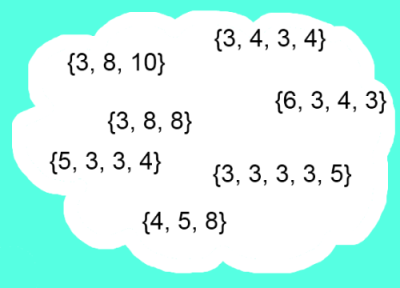#### You may also like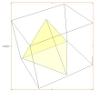### A Mean Tetrahedron

Can you number the vertices, edges and faces of a tetrahedron so that the number on each edge is the mean of the numbers on the adjacent vertices and the mean of the numbers on the adjacent faces?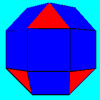### Rhombicubocts

Each of these solids is made up with 3 squares and a triangle around each vertex. Each has a total of 18 square faces and 8 faces that are equilateral triangles. How many faces, edges and vertices does each solid have?### Icosian Game

This problem is about investigating whether it is possible to start at one vertex of a platonic solid and visit every other vertex once only returning to the vertex you started at.

# Which Solids Can We Make?

##### Age 11 to 14Challenge Level

Which Solids Can We Make printable sheet
Which Solids Can We Make printable sheet - extension

This problem follows on from Semi-regular Tessellations.

Here are pictures of the five Platonic Solids - solids made from just one type of polygon, with the same number of polygons meeting at each vertex:
tetrahedron, cube, octahedron, dodecahedron, icosahedron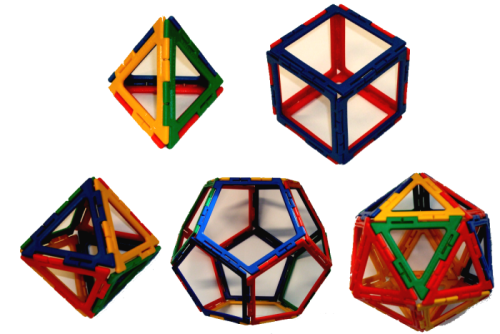Can you convince yourself that there are no more?

The angle deficit at a vertex of a polyhedron is a measure of how far short each angle sum is from $360^{\circ}$.
For example, in a dodecahedron, three pentagons with interior angles of $108^{\circ}$ meet at each vertex, so the angle sum is $324^{\circ}$ and the angle deficit is $36^{\circ}$: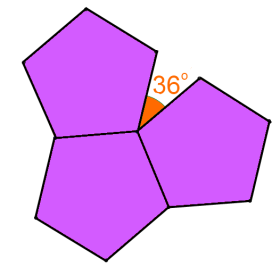Can you work out the angle deficit at the vertices of the other Platonic solids?
The total angle deficit is the sum of the deficits at each vertex.
What do you notice about the total angle deficit for the Platonic solids?

Archimedean Solids have two properties:

• They are formed by two or more types of regular polygons, each with the same side length
• Each vertex has the same pattern of polygons around it.

Here is a picture of an Archimedean Solid with 24 vertices. Its vertex form {3, 3, 3, 3, 4} is defined by the polygons that meet at each vertex: triangle, triangle, triangle, triangle, square.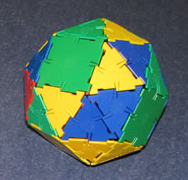Calculate the total angle deficit for this solid. Does it match your observations about the Platonic solids?

Try to suggest some other vertex forms which might give rise to Archimedean solids, assuming all solids share the property you have discovered. If you have access to construction sets such as Polydron, you could test out your ideas.

Below are some vertex forms you might like to try: some of them give rise to solids and some of them don't. Can you decide which will work before testing them out?